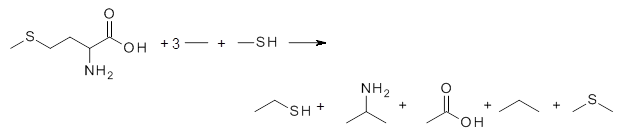Suppose you are looking at the reaction aA + bB → cC + dD. You can compute each of these molecules at two computational levels; lets call these M1 and M2. Then the reaction energy is

ΔEM1 = cEM1(C) + dEM1(D) – aEM1(A) – bEM1(B)
ΔEM2 = cEM2(C) + dEM2(D) – aEM2(A) – bEM2(A)

Now, if the two computational methods are reasonably complete, then ΔEM1 ≈ ΔEM2. This can also be true if the reaction has been selected such that one might expect very good cancellation of errors. In this case, the overall problems in computing the reactants are similar to the problems computing the products, and so these problems (i.e., errors) will cancel off. So, if we have the latter condition (a reaction constructed to obtain excellent cancellation of errors), then we might be able to exploit this idea in order to obtain energies of large molecules with a large method while avoiding to actually have to do these very large computations!

How does this work? Let’s suppose the largest molecule in the reaction is molecule C. Since

ΔEM1 ≈ ΔEM2

then

cEM1(C) + dEM1(D) – aEM1(A) – bEM1(B) ≈ cEM2(C) + dEM2(D) – aEM2(A) – bEM2(B)

cEM1(C) ≈ cEM2(C) + d[EM2(D) – EM1(D)] – a[EM2(A) – EM1(A)] – b[EM2(B) – EM1(B)]

and so

cEM1(C) ≈ cEM2(C) + Σ ci(EM2(i) – EM1(i))

So, we can get the energy of the big molecule C at the big method M1 by computing the energy of the big molecule C at the smaller method M2 along with computing all of the other molecules at both levels. If these other molecules are significantly smaller than molecule C, there can be considerable time savings here. This is the idea presented in a recent article by Raghavachari.1

The key element here is a systematic means for generating appropriate reactions, ones that (a) involve small molecules other than the molecule of interest and (b) get good cancellation of errors. Raghavachari comes up with a systematic way of creating a reaction with ever larger reference molecules. This is analogous with the methodology presented by Wheeler, Schleyer and Allen.2 Basically, the method decomposes the molecule of interest into smaller molecules that preserve the immediate chemical environment around each heavy atom, a method they call CBH-2 (connectivity-based hierarchy). The reaction below is an example of the CBH-2 decomposition reaction for methionine. (Note that CBH-2 is essentially a homodesmotic reaction and CBH-3 is essentially the group equivalent reaction I defined years ago.3)They apply the concept towards computing the energy of larger molecules (having 6-13 heavy atoms) at CCSD(T)/6-31+G(d,p) by only having to compute these large molecules at MP2/6-31+G(d,p). For a set of 30 molecules, the error in the energy of the extrapolated energy vs. the actual CCSD(T) energy is 0.35 kcal mol-1.

One of the advantages of this approach is that the small molecules are used over and over again, but they need be computed only twice, once at CCSD(T) and once at MP2.

This is certainly an approach that has been implicitly employed by many people for a long time, but here is made explicit and points towards ways to apply it even more widely.

### References

(1) Ramabhadran, R. O.; Raghavachari, K. "Extrapolation to the Gold-Standard in Quantum Chemistry: Computationally Efficient and Accurate CCSD(T) Energies for Large Molecules Using an Automated Thermochemical Hierarchy," J. Chem. Theor. Comput. 2013, ASAP DOI: 10.1021/ct400465q.

(2) Wheeler, S. E.; Houk, K. N.; Schleyer, P. v. R.; Allen, W. D. “A Hierarchy of Homodesmotic Reactions for Thermochemistry,” J. Am. Chem. Soc. 2009, 131, 2547-2560, DOI: 10.1021/ja805843n.

(3) Bachrach, S. M. “The Group Equivalent Reaction: An Improved Method for Determining Ring Strain Energy,” J. Chem. Ed. 1990, 67, 907-908, DOI: 10.1021/ed067p90.